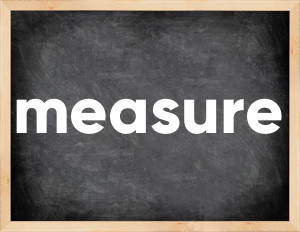# Measure past tenseThe English verb 'measure' is pronounced as [ˈmeʒər].
Related to: regular verbs.
3 forms of verb measure: Infinitive (measure), Past Simple - (measured), Past Participle - (measured).

## Here are the past tense forms of the verb measure

👉 Forms of verb measure in future and past simple and past participle.
❓ What is the past tense of measure.

## Measure: Past, Present, and Participle Forms

Base Form Past Simple Past Participle
measure [ˈmeʒər]

measured [ˈmeʒərd]

measured [ˈmeʒərd]

## What are the 2nd and 3rd forms of the verb measure?

🎓 What are the past simple, future simple, present perfect, past perfect, and future perfect forms of the base form (infinitive) 'measure'?

### Learn the three forms of the English verb 'measure'

• the first form (V1) is 'measure' used in present simple and future simple tenses.
• the second form (V2) is 'measured' used in past simple tense.
• the third form (V3) is 'measured' used in present perfect and past perfect tenses.

## What are the past tense and past participle of measure?

The past tense and past participle of measure are: measure in past simple is measured, and past participle is measured.

### What is the past tense of measure?

The past tense of the verb "measure" is "measured", and the past participle is "measured".

### Verb Tenses

Past simple — measure in past simple measured (V2).
Future simple — measure in future simple is measure (will + V1).
Present Perfect — measure in present perfect tense is measured (have/has + V3).
Past Perfect — measure in past perfect tense is measured (had + V3).

### measure regular or irregular verb?

👉 Is 'measure' a regular or irregular verb? The verb 'measure' is regular verb.

## Examples of Verb measure in Sentences

•   We have measured ozone levels since 1995 (Present Perfect)
•   Measure this and report to me (Present Simple)
•   She measures her height every day (Present Simple)
•   My grandmother is measuring pulse at the moment (Present Continuous)
•   He measures success in money (Present Simple)
•   She measures her weight daily (Present Simple)
•   I haven't measured my height since childhood (Present Perfect)